Chapter 13 Class 10 Surface Areas and Volumes

Class 10
Important Questions for Exam - Class 10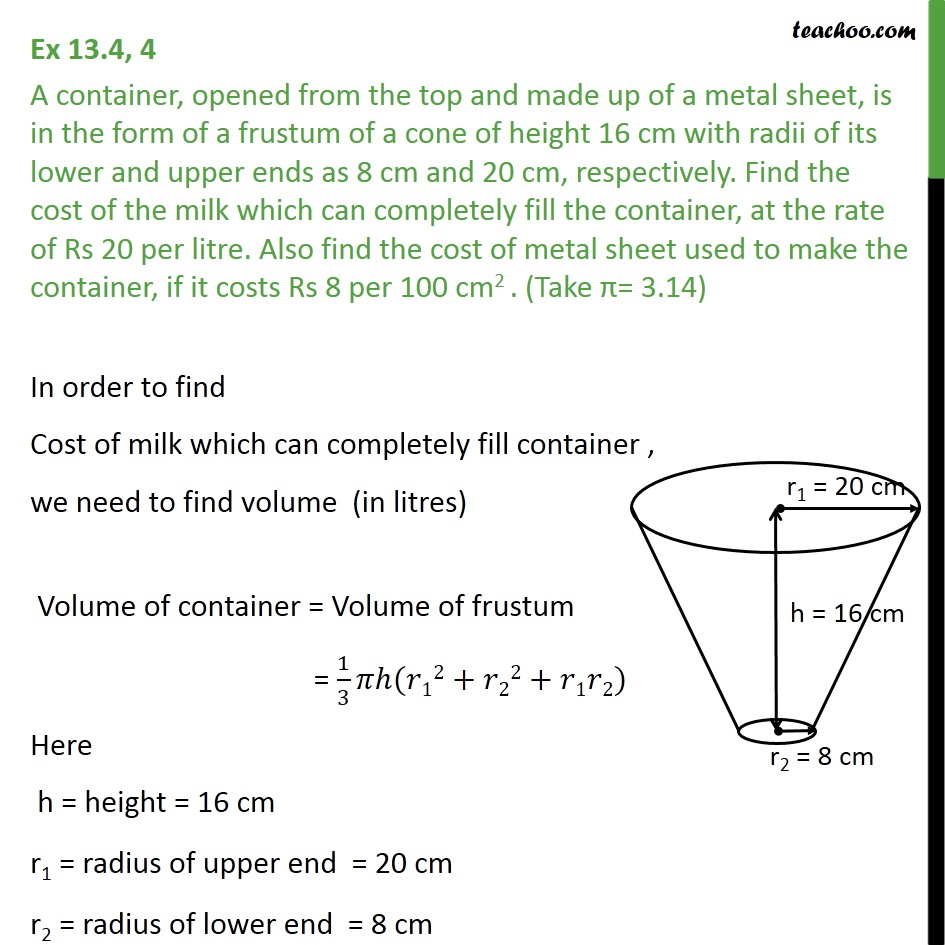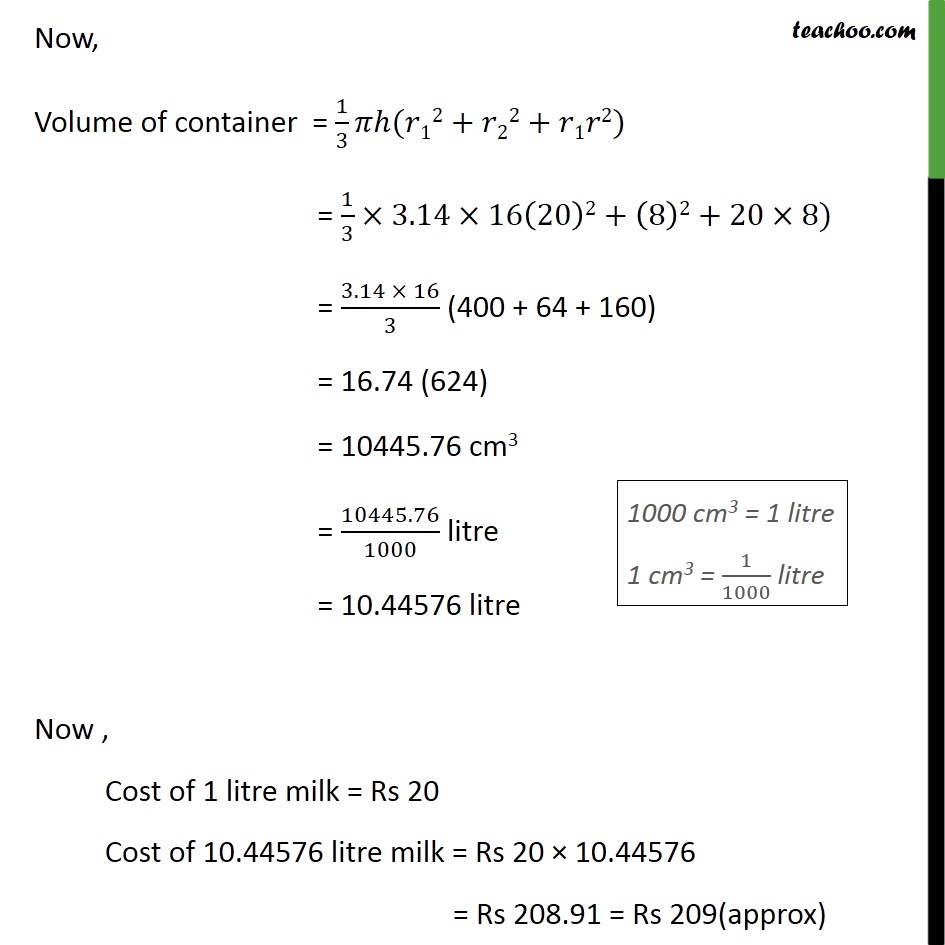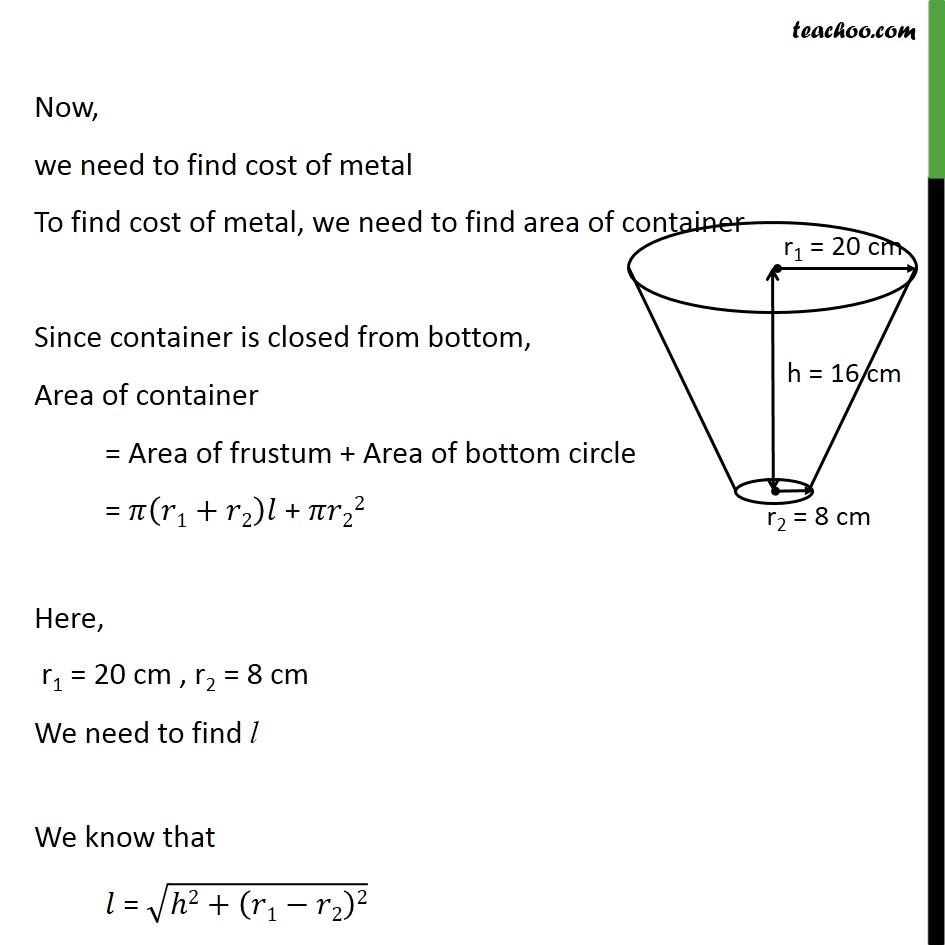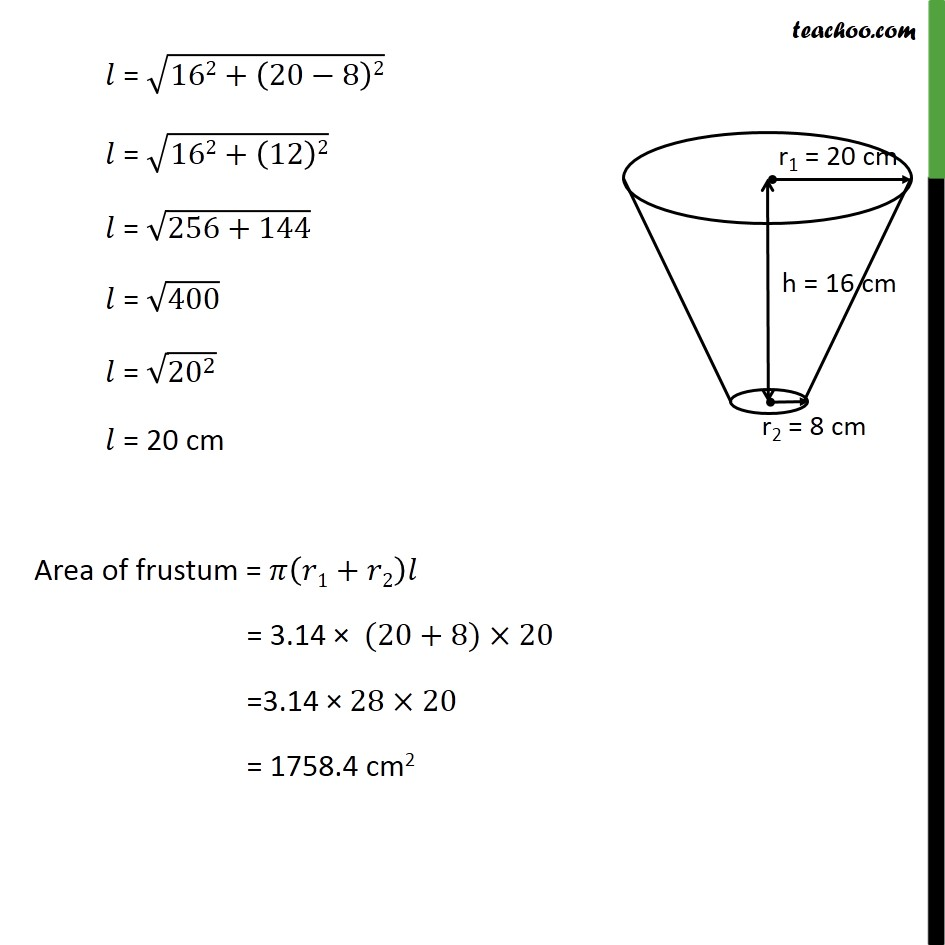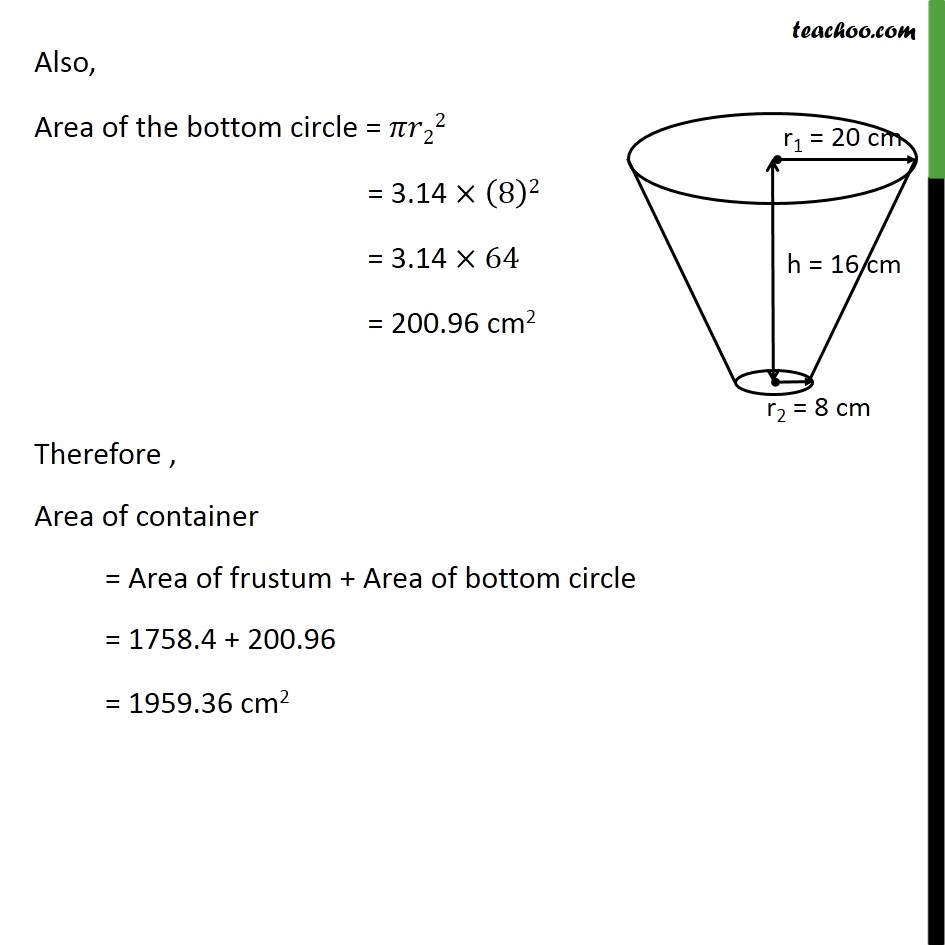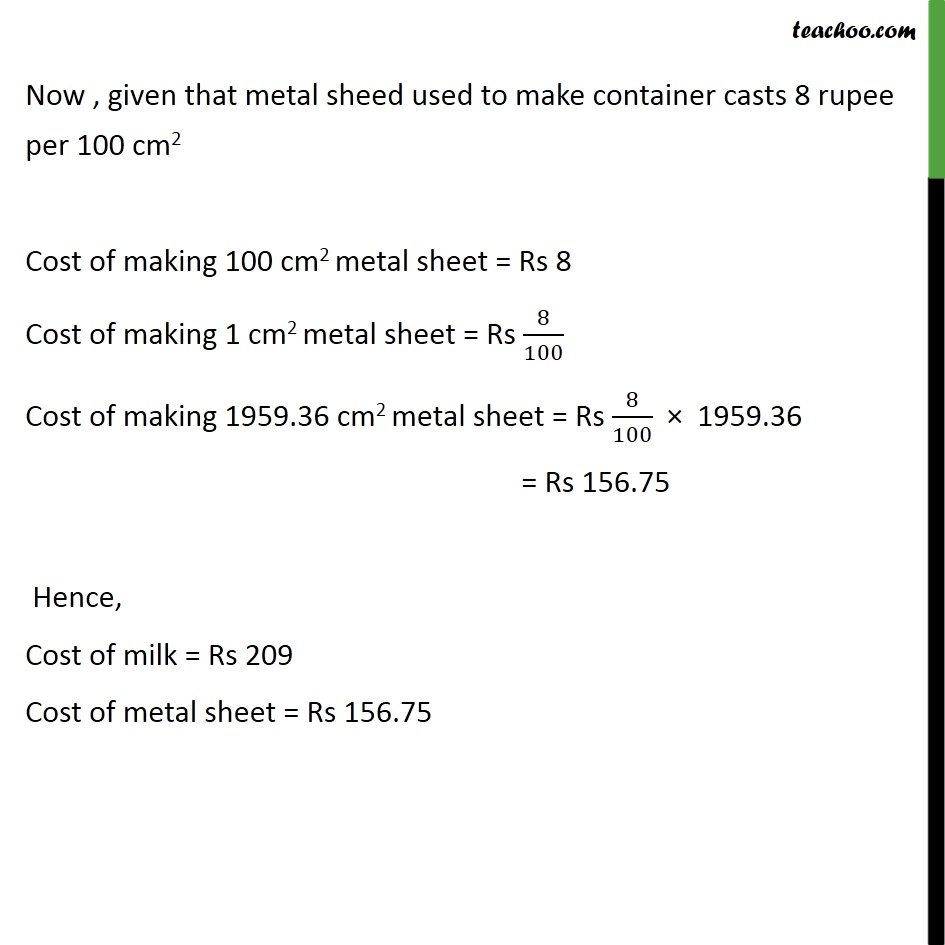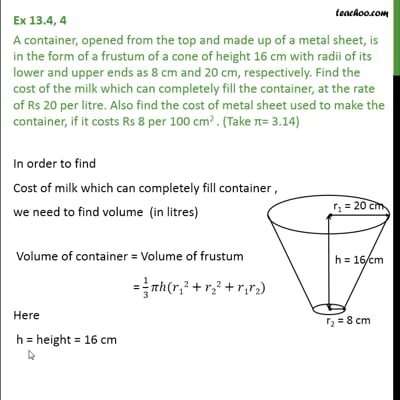This video is only available for Teachoo black users

Introducing your new favourite teacher - Teachoo Black, at only ₹83 per month

### Transcript

Ex 13.4, 4 A container, opened from the top and made up of a metal sheet, is in the form of a frustum of a cone of height 16 cm with radii of its lower and upper ends as 8 cm and 20 cm, respectively. Find the cost of the milk which can completely fill the container, at the rate of Rs 20 per litre. Also find the cost of metal sheet used to make the container, if it costs Rs 8 per 100 cm2 . (Take = 3.14) In order to find Cost of milk which can completely fill container , we need to find volume (in litres) Volume of container = Volume of frustum = 1/3 ( 12+ 22+ 1 2) Here h = height = 16 cm r1 = radius of upper end = 20 cm r2 = radius of lower end = 8 cm Now, Volume of container = 1/3 ( 12+ 22+ 1 2) = 1/3 3.14 16(20)2+(8)2+20 8) = (3.14 16)/3 (400 + 64 + 160) = 16.74 (624) = 10445.76 cm3 = 10445.76/1000 litre = 10.44576 litre Now , Cost of 1 litre milk = Rs 20 Cost of 10.44576 litre milk = Rs 20 10.44576 = Rs 208.91 = Rs 209(approx) Now, we need to find cost of metal To find cost of metal, we need to find area of container Since container is closed from bottom, Area of container = Area of frustum + Area of bottom circle = ( 1+ 2) + 22 Here, r1 = 20 cm , r2 = 8 cm We need to find l We know that = ( 2+( 1 2)2) = (162+(20 8)2) = (162+(12)2) = (256+144) = 400 = ( 20 ^2 ) = 20 cm Area of frustum = ( 1+ 2) = 3.14 (20+8) 20 =3.14 28 20 = 1758.4 cm2 Also, Area of the bottom circle = 22 = 3.14 (8)2 = 3.14 64 = 200.96 cm2 Therefore , Area of container = Area of frustum + Area of bottom circle = 1758.4 + 200.96 = 1959.36 cm2 Now , given that metal sheed used to make container casts 8 rupee per 100 cm2 Cost of making 100 cm2 metal sheet = Rs 8 Cost of making 1 cm2 metal sheet = Rs 8/100 Cost of making 1959.36 cm2 metal sheet = Rs 8/100 1959.36 = Rs 156.75 Hence, Cost of milk = Rs 209 Cost of metal sheet = Rs 156.75